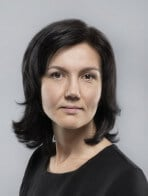# Tetiana Manzhos

Ph.D., Data Scientist, Associate Professor of Mathematics

Biography

Tetiana is a Data Scientist in Ciklum and 15 years of experienced Associate Professor of Mathematics (Courses taught: Mathematics for Economists, Theory of Probability, Quantitative Methods for Finance) in Vadym Hetman Kyiv National Economic University.

Strong education professional skilled in Mathematics. Areas of expertise: Statistical Analysis, Data Visualization, Probability Theory, and Fuzzy Logic, Linear Algebra, Mathematical Analysis, Numerical Analysis, Machine Learning Algorithms, Mathematical Modeling.

Ph.D., Institute of Mathematics NAS of Ukraine.

Taras Shevchenko National University of Kyiv, Faculty of Mechanics and Mathematics.

Preparatory course

Mathematics and Statistics for Data Science

Data Science and Analytics are mostly about learning and picking up knowledge from data. With rigorous math background, you will be able to pick up intuitions and techniques very fast, accurately and can understand how algorithms work under the hood. Standard analytics tools like linear regression, decision trees or hypothesis testing have different levels of mathematical needs but anyway they require a background in both logic and quantification, or generally math.

This course provides both mathematics and statistics as a solid background to better understand different machine learning techniques and approaches to intelligent data analysis. To demonstrate ways for solving math and stats problems with computers the Python libraries are used. We will write programs that take numbers, data or formulas as input, do the calculations needed, and then make the solutions or visualizations.